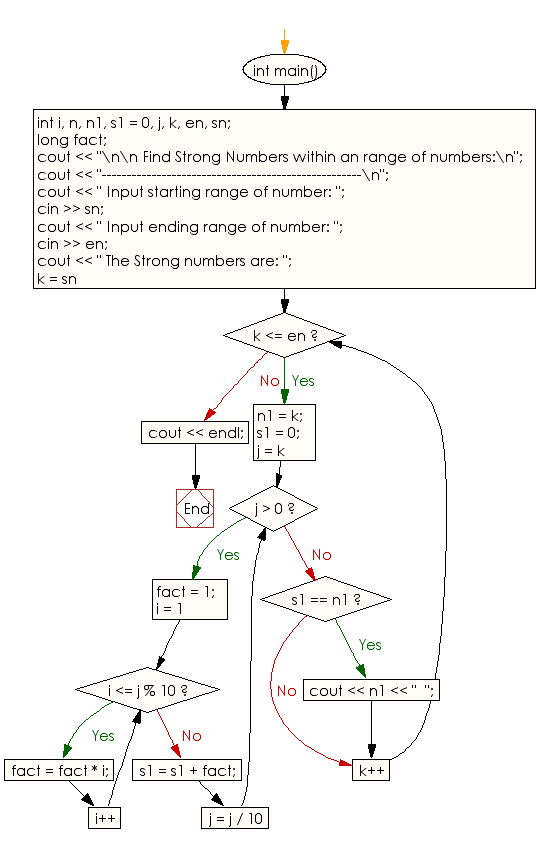﻿ C++ Exercises: Find Strong Numbers within a range of numbers - w3resource

# C++ Exercises: Find Strong Numbers within a range of numbers

## C++ Numbers: Exercise-40 with Solution

Write a C++ program to find Strong Numbers within a range of numbers.

Sample Solution:

C++ Code:

``````#include <iostream>
using namespace std;

int main()
{
int i, n, n1, s1 = 0, j, k, en, sn;
long fact;
cout << "\n\n Find Strong Numbers within an range of numbers:\n";
cout << "----------------------------------------------------\n";
cout << " Input starting range of number: ";
cin >> sn;
cout << " Input ending range of number: ";
cin >> en;
cout << " The Strong numbers are: ";
for (k = sn; k <= en; k++)
{
n1 = k;
s1 = 0;
for (j = k; j > 0; j = j / 10)
{
fact = 1;
for (i = 1; i <= j % 10; i++)
{
fact = fact * i;
}
s1 = s1 + fact;
}
if (s1 == n1)
cout << n1 << "  ";
}
cout << endl;
}
``````

Sample Output:

```Check whether a number is Strong Number or not:
-------------------------------------------------------
Input starting range of number: 1
Input ending range of number: 500
The Strong numbers are: 1  2  145
```

Flowchart:C++ Code Editor: